StatLect

# Estimation of the mean

Mean estimation is a statistical inference problem in which a sample is used to produce a point estimate of the mean of an unknown distribution.

The problem is typically solved by using the sample mean as an estimator of the population mean.

In this lecture, we present two examples, concerning:

1. normal IID samples;

2. IID samples that are not necessarily normal.

For each of these two cases, we derive the expected value, the variance and the asymptotic properties of the mean estimator.## Normal IID samples

In this example of mean estimation, which is probably the most important in the history of statistics, the sample is drawn from a normal distribution.

Specifically, we observe the realizations ofindependent random variables, ...,, all having a normal distribution with unknown meanand variance.

### The estimator

As an estimator of the mean, we use the sample mean### Expected value of the estimator

The expected value of the estimatoris equal to the true mean.

This can be proved by using the linearity of the expected value: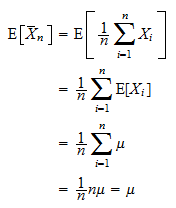Therefore, the estimatoris unbiased.

### Variance of the estimator

The variance of the estimatoris equal to.

This can be proved by using the formula for the variance of an independent sum: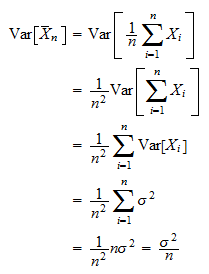Therefore, the variance of the estimator tends to zero as the sample sizetends to infinity.

### Distribution of the estimator

The estimatorhas a normal distribution:Proof

Note that the sample meanis a linear combination of the normal and independent random variables(all the coefficients of the linear combination are equal to). Therefore,is normal because a linear combination of independent normal random variables is normal. The mean and the variance of the distribution have already been derived above.

### Risk of the estimator

The mean squared error of the estimator is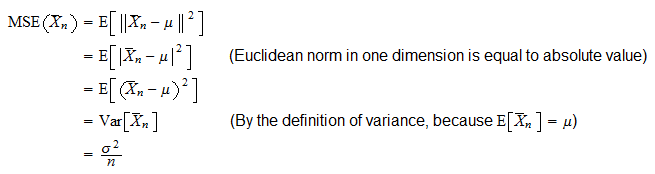### Consistency of the estimator

The sequenceis an IID sequence with finite mean.

Therefore, it satisfies the conditions of Kolmogorov's Strong Law of Large Numbers.

Hence, the sample meanconverges almost surely to the true mean:that is, the estimatoris strongly consistent.

The estimator is also weakly consistent because almost sure convergence implies convergence in probability:## IID samples

In this example of mean estimation, we relax the previously made assumption of normality.

The sample is made of the realizations ofindependent random variables, ...,, all having the same distribution with meanand variance.

### The estimator

Again, the estimator of the meanis the sample mean:### Expected value of the estimator

The expected value of the estimatoris equal to the true mean:Therefore, the estimator is unbiased.

The proof is the same found in the previous example.

### Variance of the estimator

The variance of the estimatorisAlso in this case the proof is the same found in the previous example.

### Distribution of the estimator

Unlike in the previous example, the estimatordoes not necessarily have a normal distribution: its distribution depends on those of the terms of the sequence.

However, we will see below thathas a normal distribution asymptotically, that is, it converges to a normal random variable whenbecomes large.

### Risk of the estimator

The mean squared error of the estimator is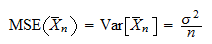The proof is the same found in the previous example.

### Consistency of the estimator

Since the sequenceis an IID sequence whose terms have finite mean, it satisfies the conditions of Kolmogorov's Strong Law of Large Numbers.

Therefore, the estimatoris both strongly consistent and weakly consistent (see example above).

### Asymptotic normality

The sequenceis an IID sequence with finite mean and variance.

Therefore, it satisfies the conditions of Lindeberg-Lévy Central Limit Theorem.

Hence, the sample meanis asymptotically normal: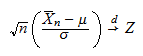whereis a standard normal random variable anddenotes convergence in distribution.

In other words, the sample meanconverges in distribution to a normal random variable with meanand variance.

## Solved exercises

Below you can find some exercises with explained solutions.

### Exercise 1

Consider an experiment that can have only two outcomes: either success, with probability, or failure, with probability.

The probability of success is unknown, but we know thatSuppose that we can independently repeat the experiment as many times as we wish and use the ratio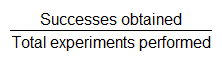as an estimator of.

What is the minimum number of experiments needed in order to be sure that the standard deviation of the estimator is less than 1/100?

Solution

Denote bythe estimator of. It can be written aswhereis the number of repetitions of the experiment andareindependent random variables having a Bernoulli distribution with parameter. Therefore,is the sample mean ofindependent Bernoulli random variables with expected valueand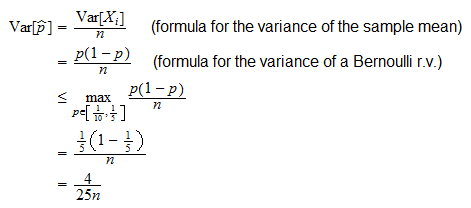ThusWe need to ensure that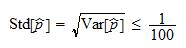orwhich is certainly verified ifor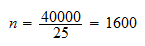### Exercise 2

Suppose that you observe a sample of 100 independent draws from a distribution having unknown meanand known variance.

How can you approximate the distribution of their sample mean?

Solution

We can approximate the distribution of the sample mean with its asymptotic distribution. So, the distribution of the sample mean can be approximated by a normal distribution with meanand variance